##### Geometry For DummiesAn angle that intersects a circle can have its vertex inside, on, or outside the circle. This article covers angles that have their vertex inside a circle—so-called chord-chord angles. The measure of a chord-chord angle is one-half the sum of the measures of the arcs intercepted by the angle and its vertical angle.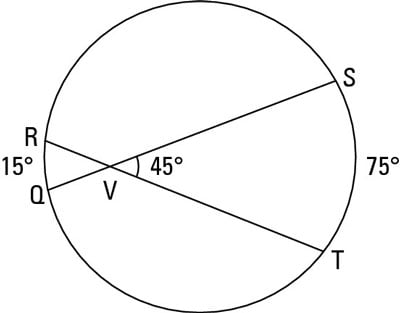For example, check out the above figure, which shows you chord-chord angle SVT. You find the measure of the angle like this: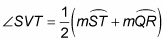Look at the following figure: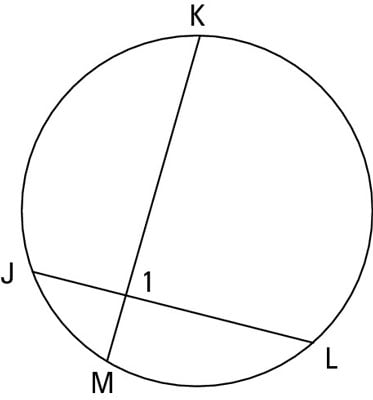Here’s a problem to show how the formula plays out: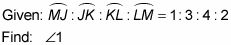To use the formula to find angle 1, you need the measures of arcs MJ and KL. You know the ratio of all four arcs is 1 : 3 : 4 : 2, so you can set their measures equal to 1x, 3x, 4x, and 2x. The four arcs make up an entire circle, so they must add up to 360°. Thus,

1x + 3x + 4x + 2x = 360

10x = 360

x = 36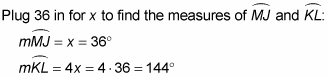Now use the formula: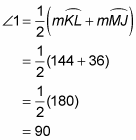That's a wrap.